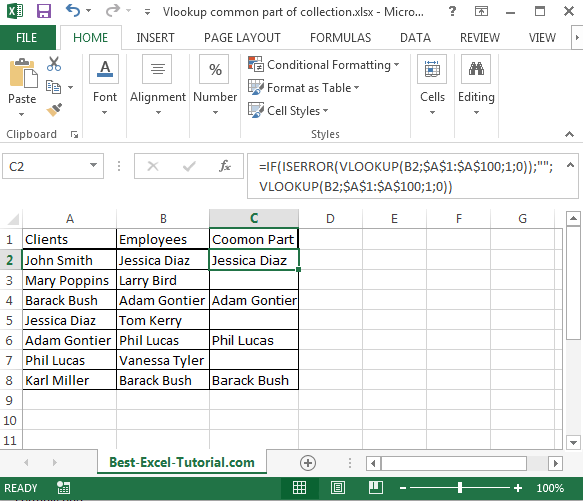#### VLOOKUP: A Guide to Usage Examples

VLOOKUP is a built-in Excel function that helps you look up a value in a table and return a corresponding value from another column. It is a very versatile function that can be used in a variety of ways.

Here are some examples of how to use VLOOKUP:

## A Simple VLOOKUP Function

This example is going to make it possible to know if the goal that has been set has been achieved in this example. We’d have a product that needed to achieve a specific goal within a certain time, and now we want to know how the sales have been on the market. This is where the VLOOKUP formula is going to be extremely useful.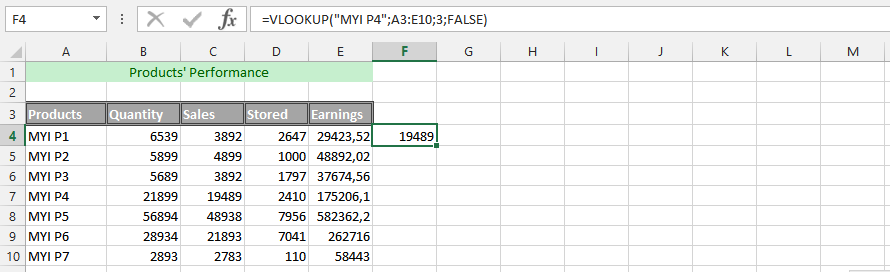## Using VLOOKUP

Twice in the same Formula In this example, we are aiming to use the VLOOKUP formulas twice, and use them to know the net worth of the sales that have been made. With the combination of the two formulas, it will make it possible to find solid details that will get us the answer that we are looking for. Let us use the same data as the one on top.

This time, we have also added another piece of data to the entire sheet, which is the total income we expect from selling the whole product. It is about knowing the performance of a specific product and comparing the total earnings with the revenues.

See also  How to use Vlookup to find a Value Greater Than a Certain Number in Excel?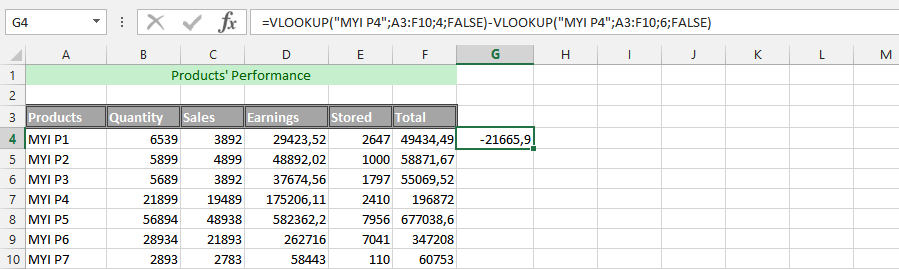This example uses the very same data as the previous one, but this time we have also set a goal for the entire product. We have already set out how much the business would be going under the circumstances that our goal has been set. We know that we want to set a specific percentage of the entire price away from the full retail price. This discount makes it possible for us to get rid of the discount that is meant to be discounted from the retail price.

For this to be successful, we need to add a new column to the data, which would be the price. This is where the formula would be useful for getting the answer that we’d like to get. We want to know how the sales will be affected.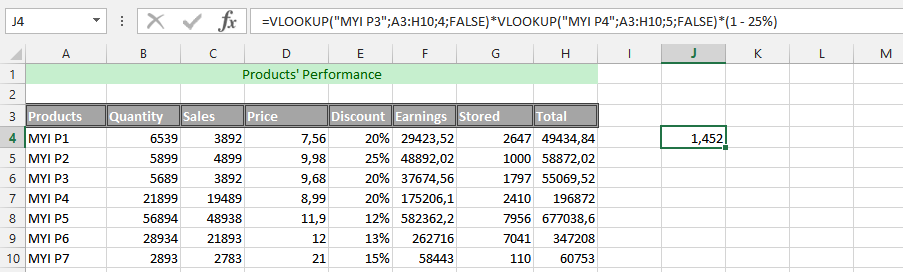## A simple IF and VLOOKUP

This is an example, where we are using the IF formula with the Vlookup formula for ease. We are using this to find out how the business is impacted by the decision, so we are using the IF and VLOOKUP formulas to find the answer we need. This is because we want to know how much the discount would be when it has been subtracted from the total earnings.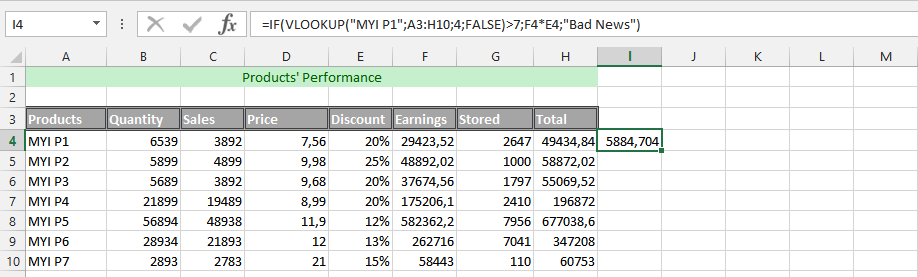## Combining a double VLOOKUP formula with an IF formula

This example is about finding out the percentage that it would cost us under the circumstances, which would be difficult for us to find out under other circumstances. The best way to do it is by finding out what the value would cost us with the discount that has been given to the products, due to the upcoming sales period.

As a result, we would want to know how this would have an effect on the revenues coming into the business as a result. This could be something that would help in acknowledging the business process.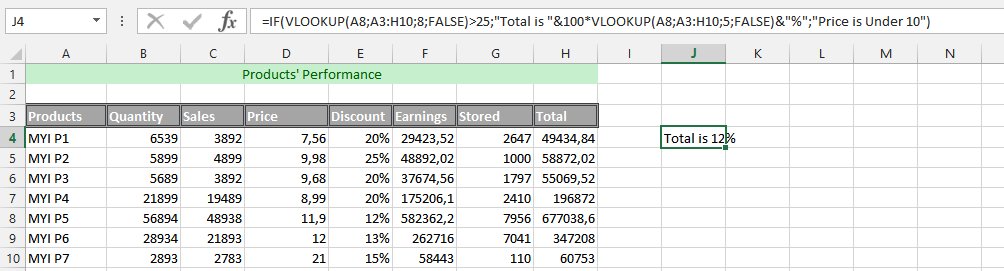## Age of Sales with Four Different Formulas

In this example, we’d have different performances, and we’d want to know the age of our sales, and determine the age. This is where four different formulas are useful in the long run, and the circumstances would require a pure understanding of simultaneously using the formulas. These formulas are INT, Yearfrac, Date and Vlookup that work together to provide the result we desire.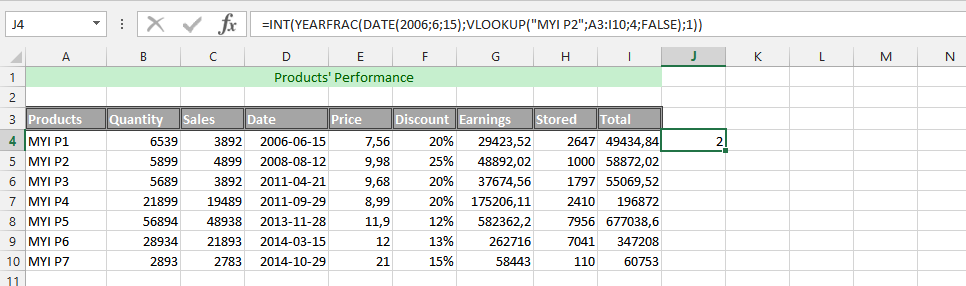## Simultaneously Using the Vlookup Function with Two Other Functions

The example is about using the IF, ISNA and VLOOKUP formulas at the same time. This is what we need to use to find the information that shows if our aim has been achieved or not. The function is based on finding solid and appropriate information that makes it possible to easily know the answer.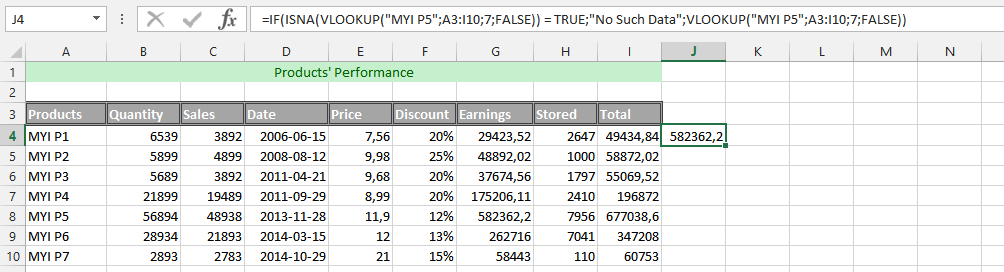## Triple VLOOKUP Formula

This example is about using three different Vlookup formulas simultaneously to find the answer that we are looking for, which would make it possible and easy to find the desired answer.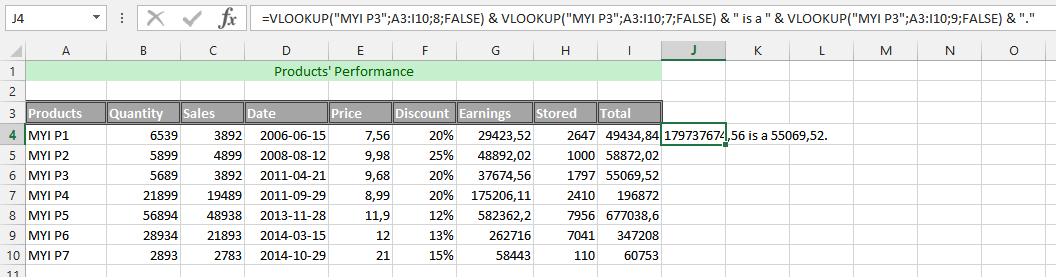## VLOOKUP with Text and Number

In this example, we are going to use the VLOOKUP formula with regard to all data being in text. We are trying to use the VLOOKUP formula to find the value that we are looking for, and under these circumstances, the whole data set has been given a label to make it successful.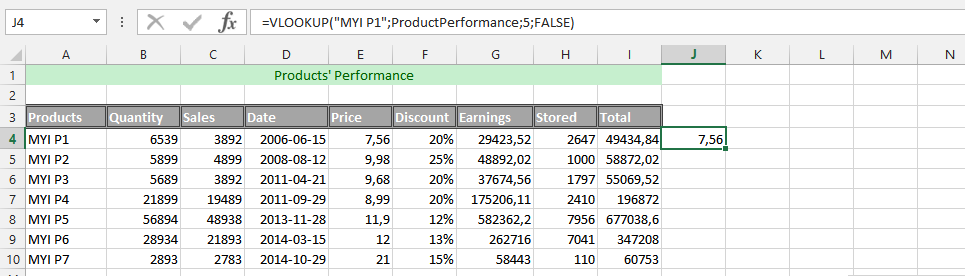## VLOOKUP from a Different Spreadsheet

This example is an excellent formula, under the circumstances that we have multiple years of experience in the business. The previous year was put into a Microsoft Excel spreadsheet, while we have the current year in another spreadsheet, but in the same document. This is where the VLOOKUP formula needs to be used with regard to the two spreadsheets.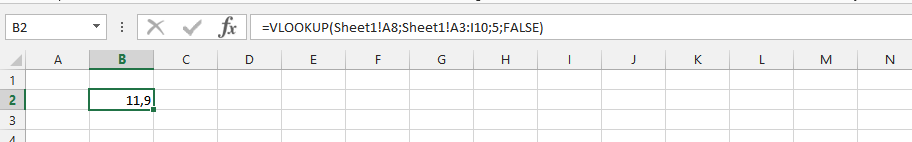## Vlookup a common part

Suppose you have two columns of data. Doesn’t matter what kind of data they contain. Let it be customers and employees. You want to find common part of these two columns.

Column A – name of your clients